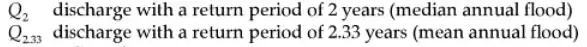# Mean Flood Return Period and QBO and ENSO

Considering the news on the extreme flooding that is occurring in South Carolina, it is interesting to consider how the mean flooding time is related to ENSO and QBO.

Handbooks on engineering hydrology specify a mean annual flood with a return period of 2.33 years. The US Geological Survey has adopted the mean annual flood Q2.33 as its standard reference value.Fig. 1 :  Definition of mean annual flood return time. From “Fluvial Forms and Processes” D. Knighton, (2014) Routledge.

The value of 2.33 years is based on years of historical records and generating cumulative histograms to best describe the statistical distribution of floods (see my book The Oil ConunDrum for many examples).Fig. 2: The distribution is characterized by a mean, median, and mode (most probable).

In this case it is related to the Gumbel probability density function shown below.  The most probable value is right around 1.5 (the peak), while the mean is 2.33 years and occurs on the downslope of the PDF.  The median describes the dividing line where half the area is to the left and half to the right.Fig. 3: Gumbel distribution used in characterizing flooding return times.

This is used in various calculations and predictions of flooding events.Fig. 2: How the mean annual flood is used in estimation. From “Handbook of Engineering Hydrology”, S. Eslamian (2014) CRC Press. The Dalrymple method dates back to 1960 and is described in this USGS report.

The essential idea of a mean in this particular case is that it gives the cycle of events if the mean value recurred periodically.  Of course the flood period is not that precisely clockwork and so a distribution of values occurs about this value. However it must have some relation to other physical events. Something has to provide enough of a buildup to release floods that are not strictly seasonal.

Even though I have not seen this cited anywhere, I propose that this value of 2.33 years is directly related to the 2.33 year fundamental forcing period seen in the Quasi-Biennial Oscillation (QBO) of stratospheric winds and of the El Nino Southern Oscillation (ENSO).  These in turn are related to periodic lunar tidal forces.

The idea is that these are periodic phenomena with definite cycle times — and thus provide a sufficient buildup and release of energy at regular intervals which will then statistically influence the flooding return periods. The impact of ENSO on flooding is obviously already significant, as occurrence of El Ninos leads to transient climate shifts in various parts of the world (the huge Australian Lake Eyre basin floods according to ENSO)It’s not that hard a concept to grasp and the fact that the fundamental periods for QBO and ENSO align with the mean flooding return time is likely not coincidental.  It would actually be hard to prove that the numbers are not related.

## One thought on “Mean Flood Return Period and QBO and ENSO”

1.admin

With the Gumbel PDF it’s very easy to generate from knowledge of the mean, median, and mode
https://en.wikipedia.org/wiki/Gumbel_distribution
Mean $\mu + \beta\,\gamma\!$
where $\gamma$ is Euler’s constant

Median $\mu – \beta\,\ln(\ln(2))\!$

Mode $\mu\!$

Like